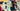# Karol Vargas

Associate Software Engineer with Mphasis# Python Grouper

May 15, 2019

## Python groupSuggester

What this program does is create a maplist of students attributes. With these attributes we assign each student a score. The way the scoring goes is that each attribute provides a different value and the higher the score the more valuable the student is to a software team. This is implemented using python and an update will be released with .csv file rather than hard coded lists.

``````import operator

name = [ "Ned Stark", "Robert Baratheon", "Jaime Lannister", "Cersei Lannister", "Daenerys Targaryen", "Jorah Mormont", "Sansa Stark", "Arya Stark", "Jon Snow", "Theon Greyjoy","Tyrion Lannister", "Stannis Baratheon", "Samwell Tarly", "Khal Drogo", "Margaery Tyrell", "Bran Stark" ]
GPA = [ 3.9,3.1,3.6,2.1,3.9,1.9,2.5,2.7,3.3,1.7,2.5,2.4,2.8,3.2,3.2,4.0]
Year = ["2019","2019","2020","2022","2019","2019","2020","2022","2021","2019","2022","2019","2019","2020","2020","2019"]
Major = ["Computer Science","Computer Science","Computer Science","Economics","Economics","Biology","Economics","Biology","Economics","Chemistry","Computer Science","Computer Science","Computer Science","Computer Science","Computer Science","Philosophy"]
# using zip() to map values
classScore = 0
mapped = zip(name, GPA, Grade, Year, Major)
# converting values to print as set
mapList = list(mapped)
len = len(list(mapped))

##create list of lists and iterate one pointer to every list inside of the list of lists. and another pointer for element  in list

def getScore(list):

#variables from maplist functions
steazlevel = 0.0
name = list
gpa = list
year = list
major = list

#gpa scoring functions
if gpa >= 3.5:
steazlevel = steazlevel + 4.0
elif gpa < 3.5 and gpa >= 2.5:
steazlevel = steazlevel + 3.0
elif gpa < 2.5 and gpa >= 1.0:
steazlevel = steazlevel + 2.0
elif gpa < 1.0:
steazlevel = steazlevel + 1.0

steazlevel = steazlevel + 4.0
steazlevel = steazlevel + 3.0
steazlevel = steazlevel + 2.0
steazlevel = steazlevel + 1.0
steazlevel = steazlevel + 0

#Year scoring functions
if year == '2019':
steazlevel = steazlevel + 1.00
elif year == '2020':
steazlevel = steazlevel + 0.75
elif year == '2021':
steazlevel = steazlevel + 0.50
elif year == '2022':
steazlevel = steazlevel + .25

#Major boolean function
if major == 'Computer Science':
steazlevel = steazlevel + 1.0

return steazlevel

def createScoreList(mapList, len):
scorelist = []
for i in range(len):
scorelist.append(getScore(mapList[i]))

return scorelist

def getList(list):

#variables from maplist functions
steazlevel = 0.0
name = list
gpa = list
year = list
major = list

#gpa scoring functions
if gpa >= 3.5:
steazlevel = steazlevel + 4.0
elif gpa < 3.5 and gpa >= 2.5:
steazlevel = steazlevel + 3.0
elif gpa < 2.5 and gpa >= 1.0:
steazlevel = steazlevel + 2.0
elif gpa < 1.0:
steazlevel = steazlevel + 1.0

steazlevel = steazlevel + 4.0
steazlevel = steazlevel + 3.0
steazlevel = steazlevel + 2.0
steazlevel = steazlevel + 1.0
steazlevel = steazlevel + 0

#Year scoring functions
if year == '2019':
steazlevel = steazlevel + 1.00
elif year == '2020':
steazlevel = steazlevel + 0.75
elif year == '2021':
steazlevel = steazlevel + 0.50
elif year == '2022':
steazlevel = steazlevel + .25

#Major boolean function
if major == 'Computer Science':
steazlevel = steazlevel + 1.0

newlist = [name, steazlevel]

return newlist
print(newlist)
print(steazlevel)
#def listtoarray(mapList, len):
#    myarray = np.asarray(StudentScore(mapList, len))
#
#    return printmyarrayprint
#def tupleCreator(mapList, len):

def getClassScore(mapList, len):
classscore = 0
for i in range(len):
classscore = classscore + getScore(mapList[i])

return classscore

def setGroupScore(inp, mapList, len):
groupScore = getClassScore(mapList, len)/inp

return groupScore

def groupSuggester(mapList, len):
count = 1
current = 0.0
for i in range(len):
current = getScore(mapList[i])

if current >= 9.0:
count = count + 1
else:
break

return count

def insertionSort(merge, len):
for i in range(1,len):

currentvalue = merge[i]
position = i

while position > 0 and merge[position-1]>currentvalue:
merge[position]=merge[position-1]
position = position-1

merge[position]=currentvalue

def setGroupOrder(list, c, len):
group = c
glist = []
for i in range(len):
glist.append(getList(mapList[i]))

return sorted(glist, key=operator.itemgetter(1), reverse=True)

def setGroup(list, c, len):
grouplist = []
groups = setGroupOrder(mapList, c, len)
# for i in range(c):
#     grouplist.append(createSublists())
#     grouplist[i] = groups[i]
length = len + 1
per_group = length / c
i = 0
g = createSublists()
for person in groups:

if i == per_group:
i = 0
grouplist.append(g)
g = createSublists()

g.append(person)
i +=1

return grouplist

def createSublists():
group = []

return group

def main():
print("Welcome to class Sorter! the best way to ensure fair groups for class projects!!!!")
print("--------------------------------------------------------------------------------")

print("class score for CS202: ")
for i in range(len):
print(getList(mapList[i]))
inp = input("How many groups Do you need for your class? ")

print("Getting Total class score...")
print(getClassScore(mapList, len))
print("\n")
print("Average score per group")
print(setGroupScore(inp, mapList, len))
print("\n")

print("Suggesting Group Size for student quality")
print("\n")
c = groupSuggester(mapList, len)
print("For the quality of students you have in the class we suggest you should have ", c," groups so everyone has the best opportunity to learn")
print("\n")

oldlist = getList(mapList)
merge = createScoreList(mapList, len)
print(merge)
print("------------------Performing insertionSort on Student Scores--------------------")
insertionSort(merge, len)
print(merge)
print("------------------insertionSort is complete. List is now sorted-----------------")

print("\n")
print(setGroup(mapList, c, len))

main()``````